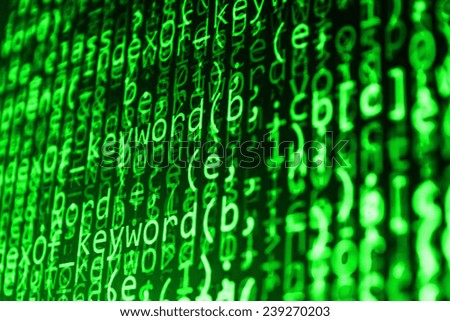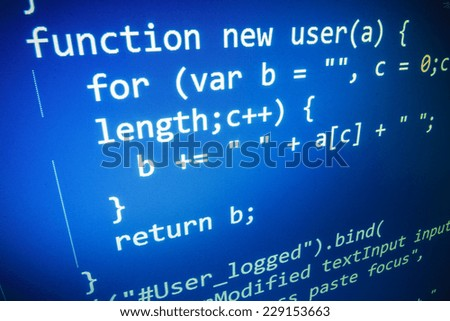## Lists

This is my

This one took me a long time to figure out how to do it, but finally here it is!

The way that I learned how to use arrays, first: I asked to Ken, then I searched in lynda.com whichever that you take it helps a lot!The most difficult part to me was that I needed to remember how to use the standard deviation, I didn't know how to translate the formula to code, and after do some drawings on my notebook I found it. And also, how to print the list of the numbers after ask it to user. Into my code are some comments that help me to remember what does each part of loop.

The only thing that I think I'm missing is the way to programm. The teacher is always telling us that we need to use functions and into "main" we only need to call those functions.

Here's my code running and the link to GitHub.## Factorial

Hi this is myTo calculate the factorial I did two codes showing two different ways to do this WSQ. The first is with loops. That is easy, I needed to declare 2 integer variables ("n" and "x") and 3 character variables (answer, yes and no). Then I putted into a do-while all my code asking for a number and do the factorial with a while loop.

Here is the code in my GitHub for this first way.

In the second code of this wsq is about recursion. This means that I did a function to calculate the factorial of a number and then, in my "main" I call that function to prints the answer.

I found this information about recursion. That helped me to understand the concept and also, there are some examples with factorial. http://www.danzig.us/cpp/recursion.html

And here is my code for this second way to do this WSQ. I hope this post will help you.

## Creation and use of strings.Hello! this is my

You can create a variable with type string in the usual ways:

`string first;`
`first = "Hello, ";`
`string second = "world.";`

The first line creates an string without giving it a value. The second line assigns it the string value "Hello." The third line is a combined declaration and assignment, also called an initialization. Normally when string values like "Hello, " or "world." appear, they are treated as C strings. In this case, when we assign them to an string variable, they are converted automatically to string values. We can output strings in the usual way:
`cout << first << second << endl;`

Also, there is a video on youtube showing this (Spanish). HERE'S THE VIDEO.

## Nested conditionals.

Hello as mi title says this post is about nested conditionals.

In addition to chaining of conditionals you can nest one conditional to another. Like in the example below:

`if (x == 0) {`

`  cout << "x is zero" << endl;`

`} `

`else {`

`  if (x > 0) {`

`    cout << "x is positive" << endl;`

`  } else {`

`    cout << "x is negative" << endl;`

`    }`

`}`

I put the code before in my editor and I compliled and run it to see how it works, I realize that actually it works!! So, we can nest conditionals into another conditionals.## Use of loops - "for"The is about more loops, and in this case I'll show you how to do a for loop. The “for loop” loops from one number to another number and increases by a specified value each time.

The syntax of the "for loop" is the next:

`for (start value; end condition; increase value){`

`  statements;`

`}`

``

This time I did a new code because I don't have used it yet. The program below print a numbers from 1 to 10.

## Use of loops - "while"is about while loop. This loop executed if the condition is TRUE, when the condition is false the loop ends.

The general syntax of this loop is the next:

`while (TRUE) {`

`  //group of statements...`

`}`

A common mistake is put a ";" at the end of the condition. DO NOT put a ";" it could cause a error.

As always I leave a example for each mastery, so here it is! of couse in my GitHub.

## Calling functions.

In we need to know and maybe teach how to call a funtions. So, people who already have done WSQ08 without copy-paste, should know how to call functions.

Functions are called by their names. If the function is without argument, it can be called directly using its name, but for functions with arguments we have two ways to call it:

1. Call by value
2. Call by reference

I can see an example? Of couse! Here in my GitHub is my WSQ08 where I use functions and I called it into main function.## Creating functions.is about how to create functions, first of all is to know what are those things?

Functions allow to structure programs in segments of code to perform individual tasks.

In C++, a function is a group of statements that is given a name, and which can be called from some point of the program. The most common syntax to define a function is:

` type name ( parameter1, parameter2, ...) { statements }`

And to show you how it works, here it is a code where I use functions and I call functions but I'll explain that in mastery11.

Keep coding! :D

## Use of "if" conditional.

is about use conditional "if". In order to write useful programs, we almost always need the ability to check
certain conditions and change the behavior of the program accordingly. Conditional
statements give us this ability. The simplest form is the if statement:

`if (x > 0) {`
`  cout << "x is positive" << endl;`
`}`

`/* if (TRUE){`

`     cout << "The condition is true" << endl;`

`   }*/`

This means that If it is true, then the statements in brackets get executed. If the condition is not true, nothing happens.

(The info before comes in the book at page 33: How to think like a computer scientist, C++ Version.)

Here is the link of my wsq05 where I used conditionals to make run the program. You can copy and paste in your editor and run it, to see how it works.## On to functions.